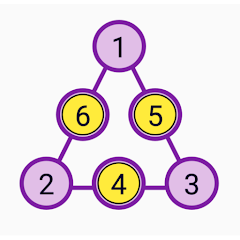# Magic Triangles - Math Puzzles

5K+Everyone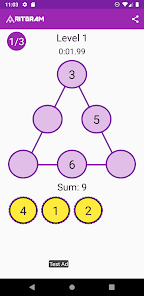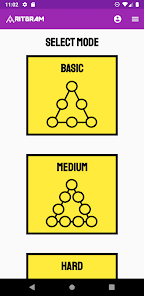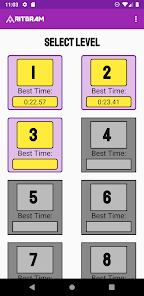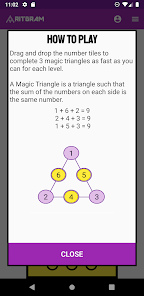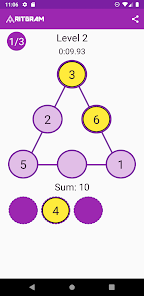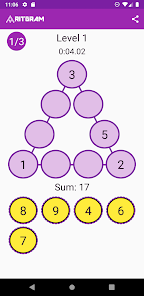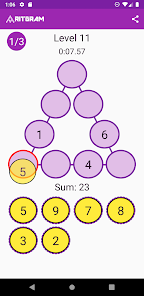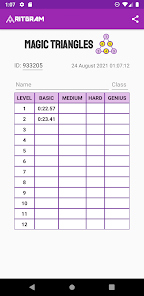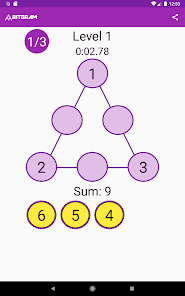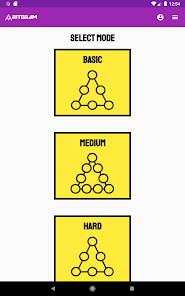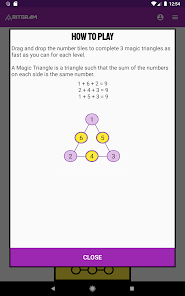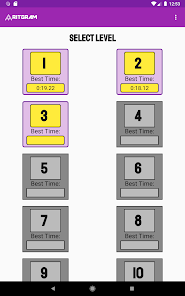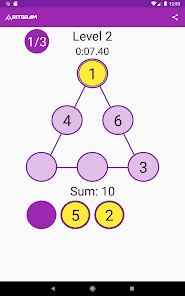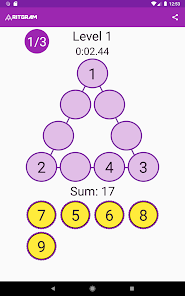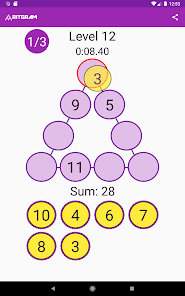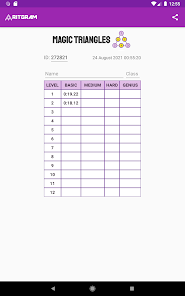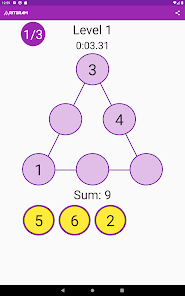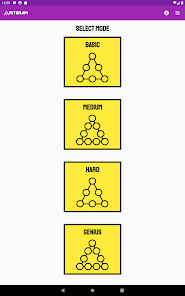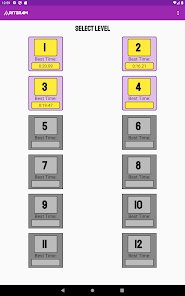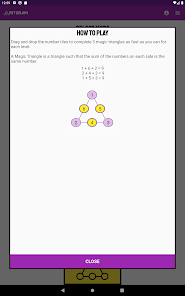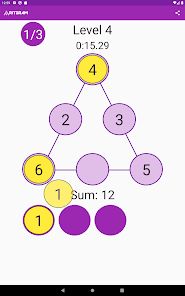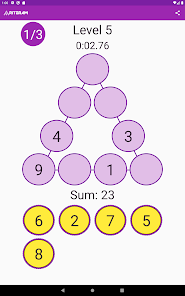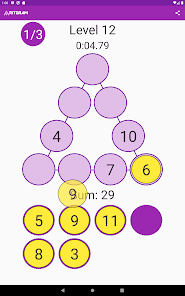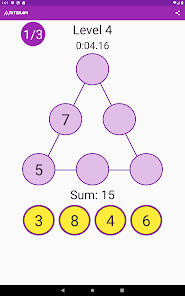Now we bring back one of the classic math puzzles, the Magic Triangles, in a fun math app. This game contains a total of 48 levels arranged in 4 difficulty modes. So put your ingenuity and mental math skills to the test, and try to solve all these magic triangles that we introduce you.

By definition, a Magic Triangle is a triangle such that the sum of the numbers on each side is the same number

The levels are arranged by difficulty. At the beginning, there are the easy levels that are useful for practicing mental math and basic operations. Later you will find magic triangles where ingenuity and numerical ability will be essential.

In this game, you have to find the tiles with the missing numbers ​​to complete the magic triangles, which you can drag and drop until you complete the puzzle. For each level you will solve 3 magic triangles. Do it as fast as you can, to put your marks at the top of the leaderboars.
Updated on
Aug 30, 2021

## Data safety